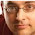## Pages

### Top 10 Greatest Moments in Math History: Primes and GIMPS

Moment 6: Primes and GIMPS
Born on September 8, 1588 in Oizé in Maine, France, Marin Mersenne discovered the formula 2^p – 1 (where p is prime) to find prime numbers . Although this formula does not find all primes, it finds what today are called the “Mersenne Primes”, the largest of which (to date), 2^77, 232,917 – 1, was found on December 26, 2017 and contains a staggering 46 million digits . The search for larger primes continues.

The Great Internet Mersenne Prime Search, or GIMPS, is a project that started in 1996 to find prime numbers with the aid of computers around the world. Anyone with a computer can take part in this project by going onto the GIMPS website and downloading the Prime95 software. Without a doubt, the founding of this project should go down as one of the top ten greatest moments in mathematical history because it completely revolutionized the science of finding prime numbers.

Before the GIMPS project, most prime searching was done by hand by finding successively larger numbers’ prime factors. All numbers have a “prime fingerprint” meaning no two numbers can be made using the same set of prime numbers multiplied. For example, the prime factors of 84 are 2, 2, 3, 7. Multiplied together, 2*2*3*7, will only ever give 84 and there is no other string of prime numbers that will multiply to get 84. This is a simple idea for small numbers, but expanded to numbers 46 million digits long is a little less easy to believe. But it’s true! Over time, mathematicians created prime factor tables to keep track of each number’s prime factors.

Factor tables were integral in finding prime numbers. Possibly the greatest success story of all time stemming from a hobby was that of J.P. Kulik’s pastime of prime factorizing numbers.

Kulik was born in 1773, died in 1863, and studied and worked at the University of Prague in the Czech Republic. Not much is recorded about this man’s life, as I could find very little about him through many internet searches. What is known is that for 20 years, Kulik factored all numbers up to the number 100 million. Although it is said that Kulik’s table had some errors, his work is still a feat incomprehensible to most. Kulik’s work was never published [4, page 116], and may be missing its second volume [10, page 335]. Born three years after Kulik’s death, American mathematician D.N. Lehmer went on to create and publish a one-volume factor table covering all numbers up to the number 10 million. It was Lehmer who made the claim that Kulik’s table had errors [4, page 116]. Even so, it’s nice to imagine a world where everyone’s hobby would become so vital to society!

Many studies have been done over the years on the nature of prime numbers and many questions still remain. In Elements, Euclid proved that there are an infinite number of prime numbers, and Peter Gustav Lejeune Dirichlet proved that for any numbers a and d, which have no common factors (are relatively prime) the sequence a, a+d, a+2d, a+3d, … will produce an infinite number of larger and larger primes [4, page 115]. Let’s try this:

Let’s look at the number 4 (2*2) and the number 15 (3*5). We can see that they are relatively prime because they have no factors in common. If a = 4 and d = 15, we have:

4, 4 +15, 4 + 2(15), 4 + 3(15), 4 + 4(15), 4 + 5(15), 4 + 6(15), 4 + 7(15) …

or

4, 19, 34, 49, 64, 79, 94, 109, …

We can see that three out of eight numbers produced from just the beginning of this sequence are in fact prime. Of course this doesn’t come even remotely close to proving the sequence finds primes, and in fact Eaves writes on page 115 of his first book Great Moments in Mathematics Before 1650 that the proof of this conjecture was quite complicated, but it does show that the sequence works for the two relatively prime numbers 4 and 15.

But what of the question of why primes seem to appear in pairs (ex: 149 and 151, 311 and 313, 821 and 823) or that every number greater than 3 can be expressed as the sum of two primes? Or what of the strange observation through the formula (An logen)/n, created by observant 15-year old, that seems to predict the density of primes between the first n positive integers where An is the number of primes less than n [4, page 116]?

Is there a pattern to primes? No one knows… yet? Maybe someday somewhere someone running GIMPS software will happen upon these unanswered questions.

Works Cited:

 Eves, Howard, Great Moments in Mathematics Before 1650, The Mathematical Association of America, 1983

 Daniell, Jessica, “Srinivasa Aiyangar Ramanujan”, University of St Andrews, Scotland, August 2005, http://www-history.mcs.st-and.ac.uk/Biographies/Ramanujan.html

 Great Internet Mersenne Prime Search (GIMPS), http://www.mersenne.org

 “Mathematics of Computation”, Vol. 29, #129, American Mathematical Society, Providence, RI, January 1975

1.1.